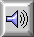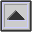GAUSSIAN NOISE or GAUSSIAN PROBABILITY DISTRIBUTION

A probability distribution describing random fluctuations in a continuous physical process; named after Karl Friedrich Gauss, an 18th century German physicist. The distribution describes such STOCHASTIC PROCESSes as the random voltage variations in a carbon resistor due to thermal motion, or the so-called Brownian motion discovered by Robert Brown, the English botanist who in 1827 first studied the rapid and apparently random motions of minute particles in a gas as seen through a microscope. The formula for the distribution implies that large deviations from the mean become less probable according to exp(-x2). It is also known as a de Moivre or normal distribution.

When an electrical variation obeys a Gaussian distribution, such as in the case of thermal motion cited above, it is called Gaussian noise, or RANDOM NOISE. Other examples occur with some types of radio tubes or semi-conductors where the noise may be amplified to produce a noise generator. Note that in all of these cases, it is only the signal's amplitude fluctuating randomly that results in its being classified as Gaussian noise. Its SPECTRUM is not necessarily similar to that of WHITE NOISE.

Compare: BACKGROUND NOISE, RUSTLE NOISE.Sound Example: Gaussian noise produced with about 4000 pulses/sec.Gaussian distribution showing the probability y of finding a deviation x from the mean (x = 0), according to the equation stated, where e is the base of natural logarithms, and s is the standard deviation. The probability of larger and larger deviations can be seen to decrease rapidly.

home